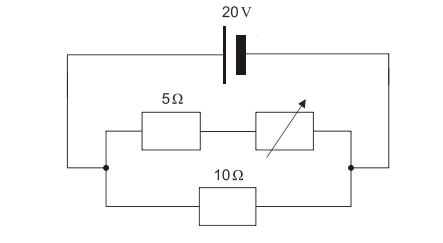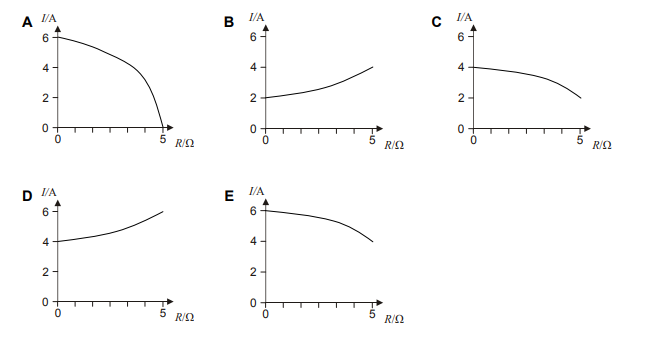# IMAT 2011 Q73 [Resistors]

Three resistors are connected to a 20V battery with a constant supply. One of the resistors is a variable resistor.The resistance of the variable resistor is gradually increased from zero to 5 Ω.
Which graph shows how the current from the battery varies with the resistance (R) of the variable resistor?2 Likes

For this question, our solution can become apparent with the calculation of 2 factors. For the sake of time management, we can choose to calculate the Current {I_t} of the battery with the end and start value of the variable resistor VR, which are 0 and 5.

So, the two questions we can now ask ourselves are:

1. When VR=0, what is {I_t} ?

2. When VR= 5, what is {I_t} ?

We can relate these two values by the equation V=IR

We can even rearrange the formula, to make I the subject; {I=\frac{V}{R}}

Since the circuit is a parallel circuit, we must note that {I_t} will inevitably split at the junction into the first branch of the circuit, where the 5 Ω. and variable resistor lay, and the second branch where the 10 Ω. resistor lays.

So we can call the current of the first branch {I_1} and the current of the second branch {I_2}.

With this is mind we also must note that after the current passes through these branches, it will rejoin again- which we can state with the formula:

{I_t}= {I_1}+ {I_2}

Now, we can begin the real calculations-

For when VR=0

Since the 5Ω resistor and the variable resistor are connected in series in the first branch, we use {R=R_1+R_2} for the final resistance of {I_1} .

For {I_1}; {R=R_1+R_2}= 5Ω+0Ω= 5Ω

{I_1=\frac{V}{R}}= {\frac{20V}{5Ω+0Ω}}= 4 A

{I_2=\frac{V}{R}}= {\frac{20V}{10Ω}}= 2 A

{I_t}= {I_1}+ {I_2}= 4A + 2A= 6A

To put this into a position on the given graphs, we see that our X-axis is the Resistance in Ohms, and our Y-axis is the Current from the battery in Amperes.

(X,Y)= (0,6)

This alone eliminates options B, C and D- and we are left to analyze A or E.

Our final step is calculating For when VR=5

For {I_1}; {R=R_1+R_2}= 5Ω+5Ω= 10Ω

{I_1=\frac{V}{R}}= {\frac{20V}{10Ω}}= 2 A

{I_2=\frac{V}{R}}= {\frac{20V}{10Ω}}= 2 A

{I_t}= {I_1}+ {I_2}= 2A + 2A= 4A

Again, we put this into a position on the given graphs, where we know that our X-axis is the Resistance in Ohms, and our Y-axis is the Current from the battery in Amperes.

(X,Y)= (5,4)

Leaving our final answer to clearly be E.

3 Likes